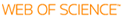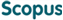BROWSE

Related ResearcherSun, Hae-sang
Number Theory Group
Research Interests
• Zeta function, L-function, mu invariant, indivisibility of special L-values

Derivative of power series attached to Gamma-transform of p-adic measures

Cited 3 times inCited 2 times inTitle
Derivative of power series attached to Gamma-transform of p-adic measures
Other Titles
Derivative of power series attached to Gamma-transform of p-adic measures
Author
Issue Date
2010-01
Publisher
Citation
JOURNAL OF NUMBER THEORY, v.130, no.1, pp.10 - 26
Abstract
We extend the result of Angles (2007) , namely f'(T; theta) not equivalent to 0 (mod p) for the Iwasawa power series f(T; theta) is an element of (Z) over bar (p)parallel to T - 1 parallel to. For the derivative D = Td/dT, a numerical polynomial Q on Z(p), and a prime pi in (Z) over bar (p) over p, we show that Q(D)f(T: theta) equivalent to 0 (mod pi) if and only if Q equivalent to 0 (mod pi) i.e. Q (x) equivalent to 0 (mod pi) for all x is an element of Z(p). This result comes from a similar assertion for the power series attached to the Gamma-transform of a p-adic measure which is related to a certain rational function in (Z) over bar (p)parallel to T - 1 parallel to. (C) 2009 Elsevier Inc. All rights reserved
URI
https://scholarworks.unist.ac.kr/handle/201301/13336
URL
http://www.sciencedirect.com/science/article/pii/S0022314X09002029
DOI
10.1016/j.jnt.2009.07.001
ISSN
0022-314X
Appears in Collections:
MTH_Journal Papers
Files in This Item:
There are no files associated with this item.